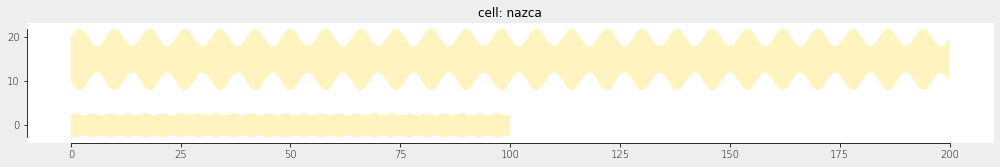Home Forums Nazca Questions and Answers Drawing line with mathematical function

Viewing 5 posts - 1 through 5 (of 5 total)
• Author
Posts
• #6047

Hello, I am very new to Nazca design and eager to learn more. I see tutorial examples showing parametric curves which have various bends using python functions. I couldn’t figure out how to draw a simple line with one parametric equation. For example, something like:

nd.sin(wavelength,amplitude,number of cycles)

sorry if this question shows a lack of effort, I’ve spent over 2 hours now trying accomplish this. If you could also include where I could have found this information myself that would great too!

Thank you!

#6049

Dear Cameron,

If I interpret your question correctly, i.e. that you want to modulate the edge of the waveguide, there are a few ways to get a sin-shaped waveguide edge.

The most flexible is to define a parametric curve in x(t), y(t), and w(t), where in your case you exploit w(t). See also Free form curves, which discusses arbitrary parametric curves.

``````import numpy as np
import nazca as nd

xs.width = 5.0

# create functions x, y, w for the parametric waveguide:
def x(t, wavelength, periods, **kwargs):
"""X as function of t and free parameters."""
return periods * wavelength * t
def y(t, **kwargs):
"""Y as function of t and free parameters."""
return 0
def w(t, width1=None, width2=None, amplitude=1.0, periods=10, **kwargs):
"""Width as function of t, width1 and width2 and free parameters."""
return width1 + amplitude * np.sin(t * periods * np.pi)

# create the new parametric function using the template Tp_viper():
params = {'wavelength': 2.0, 'amplitude': 0.5, 'periods': 50}  # assign *all* free params used in functions x, y, and w.
sin_edge = nd.Tp_viper(x, y, w, xs='myXS', **params)

# put waveguides:
sin_edge().put(0)
sin_edge(width1=10.0, wavelength=4.0, amplitude=4.0, N=2000).put(0, 15)
nd.export_gds()``````

The result is the following waveguides:The second solution is to define a perturbation of the edge of a straight waveguide element. This hasn’t been an actively in use option, but I dusted it off for nazca-0.5.10 to work in the following way (not using a sin but 2*t for simplicity). The edge1 function in t adds to the normal width of the guide, but it becomes absolute when using width=1.0.

``````import nazca as nd
s = nd.strt(length=10, edge1=lambda t: 2*t + 1.0, edgepoints=100, layer=1).put(0)
nd.export_gds()``````

The above would work the same for straight interconnects.

Lastly, you can draw your desired waveguide directly as a polygon:

``````import nazca as nd
points = [(x1, y1), (x2, y2), ...] # your shape
nd.Polygon(points=points, layer=1).put(0)
nd.export_gds()``````

You ideally would put this in a cell and add pins to it as described in Make and put a Cell.

Ronald

#6050

Thanks for your help Ronald! This was very useful and interesting, I will refer to this script in the future. However, I am also wondering not only about the sidewall shape of the waveguide, but rather the path of the waveguide itself. A separate question is how do I measure the total path length of a waveguide made with Nazca design? In the case I can create the waveguide path with a mathematical function, I can analytically derive the path length, however I still didn’t figure that out, and it wouldn’t work in general. Thanks again for your advice!

Best,

Cameron

#6051

Actually, as I think more about your script, I guess modifying the waveguide edge and the path length are kind of one in the same thing . Essentially I only need to modify your script by putting the top and bottom waves (which define the top and bottom edges) out of phase by 90 degrees from each other, then it would create a uniform line width which follows a sinusoidal path. I could analytically derive the path length from there. However, I couldn’t find the function to obtain path length in general. Thanks again.

#6052

Dear Cameron,

A correct observation; You can play with x, y, and w at the same time to get what you need.

For the geometrical path length of a parametric curve along (x, y) some calculus is required:

length = integral [ sqrt (x(t)^2 + y(t)^2) * dt ], t from t=0 to t=1

Analytically you may have the integral already, then that would be the most accurate solution. Otherwise take the sum of the line segments for a set of finite dt sections for t along [0, 1], where you need to set a criterion that ensures you obtain a sufficiently close approximation of the length. Perhaps scipy.integrate is of use.

Ronald

Viewing 5 posts - 1 through 5 (of 5 total)
• You must be logged in to reply to this topic.